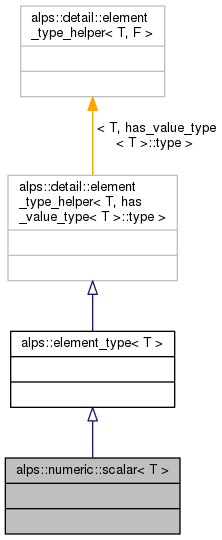ALPSCore reference
alps::numeric::scalar< T > Struct Template Reference

Metafunction returning "mathematical scalar" type for type T. More...

#include <scalar.hpp>

Inheritance diagram for alps::numeric::scalar< T >:Collaboration diagram for alps::numeric::scalar< T >:## Detailed Description

### template<typename T> struct alps::numeric::scalar< T >

Metafunction returning "mathematical scalar" type for type T.

The mathematical scalar is defined as type that can be used to scale the value of type T. Note that it is not necessarily any underlying storage type for the object of type T!

Definition at line 28 of file scalar.hpp.

The documentation for this struct was generated from the following file: